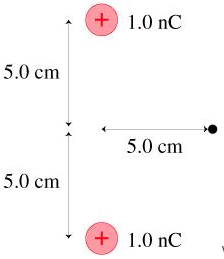# Problem: (a) What is the strength of the electric field at the position indicated by the dot in the figure?(b) What is the direction of the electric field at the position indicated by the dot in the figure? Specify the direction as an angle above the horizontal line.

###### FREE Expert Solution

The electric field is caused by a charged body.

(a) Strength of the electric field:

$\overline{){\mathbf{E}}{\mathbf{=}}\frac{\mathbf{k}\mathbf{·}\mathbf{q}}{{\mathbf{r}}^{\mathbf{2}}}}$, where

•  k = 8.988×109 N•m2/C(Coulomb's constant).
• q - charge of the particle, and
• r - the distance from the charged particle.
83% (389 ratings)###### Problem Details

(a) What is the strength of the electric field at the position indicated by the dot in the figure?
(b) What is the direction of the electric field at the position indicated by the dot in the figure? Specify the direction as an angle above the horizontal line.